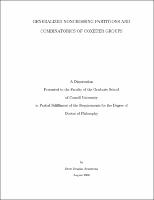## Generalized noncrossing partitions and combinatorics of Coxeter groupsThis thesis serves two purposes: it is a comprehensive introduction to the Catalan combinatorics'' of finite Coxeter groups, suitable for nonexperts, and it also introduces and studies a new generalization of the poset of noncrossing partitions. This poset is part of a Fuss-Catalan combinatorics'' of finite Coxeter groups, generalizing the Catalan combinatorics. Our central contribution is the definition of a generalization $NC^{(k)}(W)$ of the poset of noncrossing partitions corresponding to each finite Coxeter group $W$ and positive integer $k$. This poset has elements counted by a generalized Fuss-Catalan number $\Cat^{(k)}(W)$, defined in terms of the invariant degrees of $W$. We develop the theory of this poset in detail. In particular, we show that it is a graded semilattice with beautiful structural and enumerative properties. We count multichains and maximal chains in $NC^{(k)}(W)$. We show that the order complex of $NC^{(k)}(W)$ is shellable and hence Cohen-Macaulay, and we compute the reduced Euler characteristic of this complex. We show that the rank numbers of $NC^{(k)}(W)$ are polynomial in $k$; this defines a new family of polynomials (called Fuss-Narayana) associated to the pair $(W,k)$. We observe some fascinating properties of these polynomials. We study the structure $NC^{(k)}(W)$ more specifically when $W$ is a classical type $A$ or type $B$ Coxeter group. In these cases, we show that $NC^{(k)}(W)$ is isomorphic to a poset of noncrossing'' set partitions in which each block has size divisible by $k$. Hence, we refer to $NC^{(k)}(W)$ in general as the poset of $k$-divisible noncrossing partitions''. In this case, we prove rank-selected and type-selected enumeration formulas for multichains in $NC^{(k)}(W)$. We also describe new bijections between multichains of classical noncrossing partitions and classical $k$-divisible noncrossing partitions. It turns out that our poset $NC^{(k)}(W)$ shares many enumerative features in common with the generalized nonnesting partitions of Athanasiadis and the generalized cluster complex of Fomin and Reading. We give a basic introduction to these topics and describe several new conjectures relating these three families of Fuss-Catalan objects''. We mention connections with the theories of cyclic sieving and diagonal harmonics.